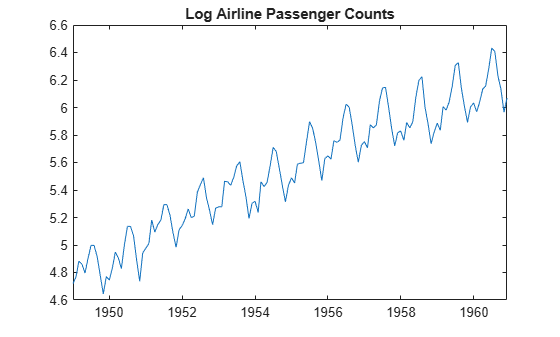# Nonseasonal and Seasonal Differencing

This example shows how to apply both nonseasonal and seasonal differencing using lag operator polynomial objects. The time series is monthly international airline passenger counts from 1949 to 1960.

Load the airline data set (`Data_Airline.mat`).

```load('Data_Airline.mat') y = log(Data); T = length(y); figure plot(y) h1 = gca; h1.XLim = [0,T]; h1.XTick = [1:12:T]; h1.XTickLabel = datestr(dates(1:12:T),10); title 'Log Airline Passenger Counts';```The data shows a linear trend and a seasonal component with periodicity 12.

Take the first difference to address the linear trend, and the 12th difference to address the periodicity. If ${y}_{t}$ is the series to be transformed, the transformation is

`$\Delta {\Delta }_{12}{y}_{t}=\left(1-L\right)\left(1-{L}^{12}\right){y}_{t},$`

where $\Delta$ denotes the difference operator, and $L$ denotes the lag operator.

Create the lag operator polynomials $1-L$ and $1-{L}^{12}$. Then, multiply them to get the desired lag operator polynomial.

```D1 = LagOp({1 -1},'Lags',[0,1]); D12 = LagOp({1 -1},'Lags',[0,12]); D = D1*D12```
```D = 1-D Lag Operator Polynomial: ----------------------------- Coefficients: [1 -1 -1 1] Lags: [0 1 12 13] Degree: 13 Dimension: 1 ```

The first polynomial, $1-L$, has coefficient 1 at lag 0 and coefficient -1 at lag 1. The seasonal differencing polynomial, $1-{L}^{12}$, has coefficient 1 at lag 0, and -1 at lag 12. The product of these polynomials is

`$\left(1-L\right)\left(1-{L}^{12}\right)=1-L-{L}^{12}+{L}^{13},$`

which has coefficient 1 at lags 0 and 13, and coefficient -1 at lags 1 and 12.

Filter the data with differencing polynomial `D` to get the nonseasonally and seasonally differenced series.

```dY = filter(D,y); length(y) - length(dY)```
```ans = 13 ```

The filtered series is 13 observations shorter than the original series. This is due to applying a degree 13 polynomial filter.

```figure plot(14:T,dY) h2 = gca; h2.XLim = [0,T]; h2.XTick = [1:12:T]; h2.XTickLabel = datestr(dates(1:12:T),10); axis tight; title 'Differenced Log Airline Passenger Counts';```The differenced series has neither the trend nor seasonal component exhibited by the original series.